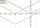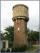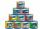Find two

Find two consecutive natural numbers whose product is 1 larger than their sum. Searched numbers expressed by a fraction whose numerator is the difference between these numbers and the denominator is their sum.

Result

a =  2
b =  3

Solution:Checkout calculation with our calculator of quadratic equations.Leave us a comment of example and its solution (i.e. if it is still somewhat unclear...):Be the first to comment!To solve this verbal math problem are needed these knowledge from mathematics:

Looking for help with calculating roots of a quadratic equation? Do you have a linear equation or system of equations and looking for its solution? Or do you have quadratic equation?

Next similar examples:Find the roots of the quadratic equation: 3x2-4x + (-4) = 0.
2. VariableFind variable P: PP plus P x P plus P = 160
3. Evaluation of expressionsIf a2-3a+1=0, find (i)a2+1/a2 (ii) a3+1/a3
4. ProductThe product of two consecutive odd numbers is 8463. What are this numbers?In six baskets, the seller has fruit. In individual baskets, there are only apples or just pears with the following number of fruits: 5,6,12,14,23 and 29. "If I sell this basket," the salesman thinks, "then I will have just as many apples as a pear." Which
6. Theorem proveWe want to prove the sentence: If the natural number n is divisible by six, then n is divisible by three. From what assumption we started?
7. Difference of two numberThe difference of two numbers is 20. They are positive integers greater than zero. The first number raised to one-half equals the second number. Determine the two numbers.
8. Equation with abs valueHow many solutions has the equation ? in the real numbers?
9. Water reservoirThe cuboid reservoir contains 1900 hectoliters of water and the water height is 2.5 m. Determine the dimensions of the bottom where one dimension is 3.2 m longer than the second one.
10. Mom and daughterMother is 39 years old. Her daughter is 15 years. For many years will mother be four times older than the daughter?
11. EquationEquation ? has one root x1 = 8. Determine the coefficient b and the second root x2.
12. DiscriminantDetermine the discriminant of the equation: ?
13. Solve 3Solve quadratic equation: (6n+1) (4n-1) = 3n2
14. RootsDetermine the quadratic equation absolute coefficient q, that the equation has a real double root and the root x calculate: ?
15. FractionFor what x expression ? equals zero?
16. CansHow many cans must be put in the bottom row if we want 182 cans arrange in 13 rows above so that each subsequent row has always been one tin less? How many cans will be in the top row?Quadratic equation ? has roots x1 = 80 and x2 = 78. Calculate the coefficients b and c.